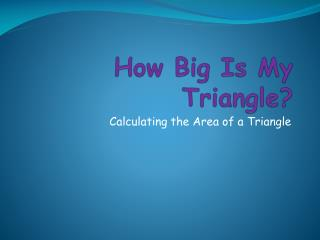DownloadDownload PresentationHow Big Is My Triangle?

# How Big Is My Triangle?

Download Presentation## How Big Is My Triangle?

- - - - - - - - - - - - - - - - - - - - - - - - - - - E N D - - - - - - - - - - - - - - - - - - - - - - - - - - -
##### Presentation Transcript

1. How Big Is My Triangle? Calculating the Area of a Triangle

2. A triangle is one-half of a parallelogram. The second triangle has been turned upside down. When the second triangle is placed adjacent to the first one, the two triangles form a parallelogram. 1st triangle 2nd triangle

3. You know how to find the area of a parallelogram. Height (h) = 4 in Base × Height (b × h) = Area 6 × 4 = 24 square inches Base (b) = 6 in

4. If a triangle is half of a parallelogram, how do you think you find the area of a triangle? Multiply base by height (b × h) Multiply base by height and divide by 2 (b × h) ÷ 2 Multiply base by height and multiply by 2 b × h × 2

5. So, how do you find the base and height of a triangle? A right triangle is easy This isosceles triangle isn’t too hard This scalene triangle can be a little more challenging height base height base height base

6. What is the area of the triangle below? Use the formula (b × h) ÷ 2 15 square inches 10 square inches 7½ square inches 5 in 3 in

7. Is the area of this triangle 8 square inches? 4 inches 4 inches

8. Draw lines to show where you could measure the base and height of this triangle.

9. If this triangle has the specified base and height, what is its area? 21 square inches 42 square inches 13½ squareinches 6 in 7 in

10. Draw lines to show where you could measure the base and height of this triangle.

11. If this triangle has the specified base and height, what is its area? 4½ square inches 18 square inches 9 square inches 9 in 2 in